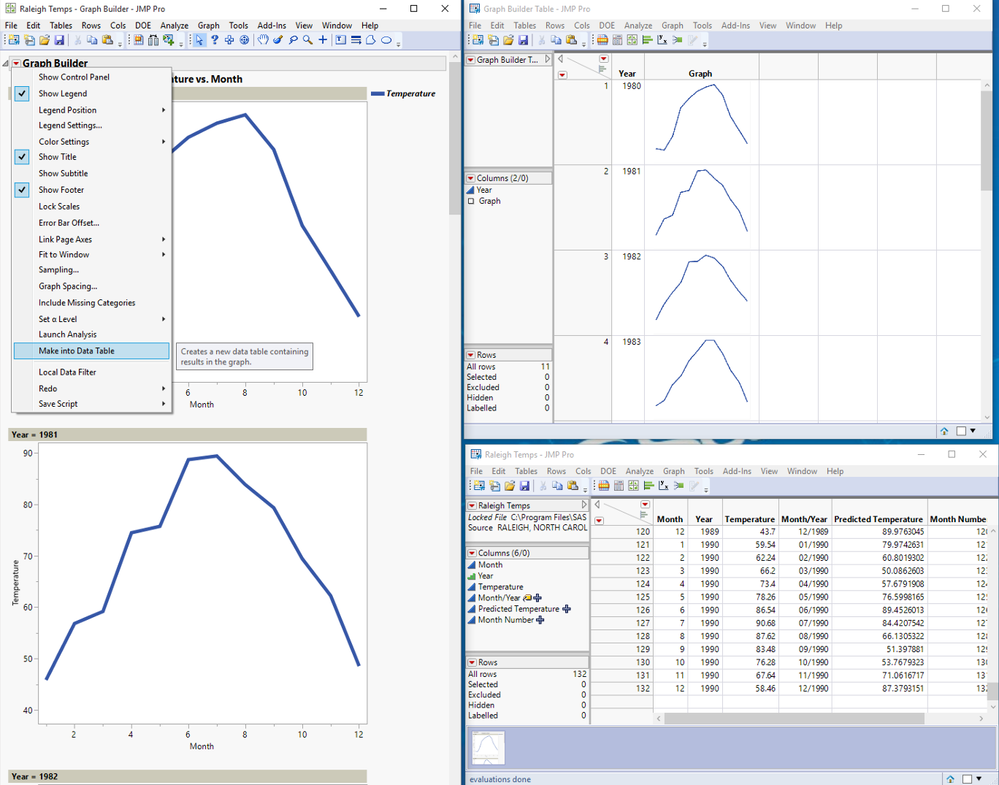Choose Language Hide Translation Bar
Highlighted

## Generating sparklines for each row in a column

What I want to do is something I have seen in one of the FDE tutorials Using JMP® Pro to Pre-Process Functional Data and Create Surrogate Models, but I can't find the code that describes how the shown data was created:

How would I create a column like X3 with my own data?

Is X3 an interactive column (e.g., can be modified or updated) or just a picture column like I have seen in other examples like the Big class families sample data?

The former would be more interesting, but I could live with the latter if necessary.

1 ACCEPTED SOLUTION

Accepted Solutions
Highlightedstan_koprowskiCommunity Manager

## Re: Generating sparklines for each row in a column

Hi @Feli,

Create a graph using graph builder.

Click the Make Into Data Table option from the red triangle to create a table of graphs.Sparklines using JMP GraphBuilder

Using the Raleigh Temps sample data table I created a line graph using Graph Builder as follows--

I changed year to ordinal from continuous so I can have a single year for each graph.

Y: Temperature

X: Month

Page By: Year

JSL Script for creating the graph--

``````Graph Builder(
Variables( X( :Month ), Y( :Temperature ), Page( :Year ) ),
Elements( Line( X, Y, Legend( 4 ) ) ),
SendToReport(
Dispatch(
{},
"400",
ScaleBox,
{Legend Model(
4,
Properties( -1, {Line Width( 4 )}, Item ID( "Mean", 1 ) )
)}
)
)
);``````

Highlightedstan_koprowskiCommunity Manager

## Re: Generating sparklines for each row in a column

Hi @Feli,

Create a graph using graph builder.

Click the Make Into Data Table option from the red triangle to create a table of graphs.Sparklines using JMP GraphBuilder

Using the Raleigh Temps sample data table I created a line graph using Graph Builder as follows--

I changed year to ordinal from continuous so I can have a single year for each graph.

Y: Temperature

X: Month

Page By: Year

JSL Script for creating the graph--

``````Graph Builder(
Variables( X( :Month ), Y( :Temperature ), Page( :Year ) ),
Elements( Line( X, Y, Legend( 4 ) ) ),
SendToReport(
Dispatch(
{},
"400",
ScaleBox,
{Legend Model(
4,
Properties( -1, {Line Width( 4 )}, Item ID( "Mean", 1 ) )
)}
)
)
);``````

Article Labels